# What is an Impedance Meter?

## What is an impedance meter? Impedance basics and key measurement considerations

### Overview

Impedance, a measure of the resistance in an alternating current (AC) circuit, is not visible. Consequently, it must be measured with a dedicated instrument known as an impedance meter. If you’ve never used an impedance meter, you may have a number of questions, for example how the instrument is used and what you need to be careful of when using it.

•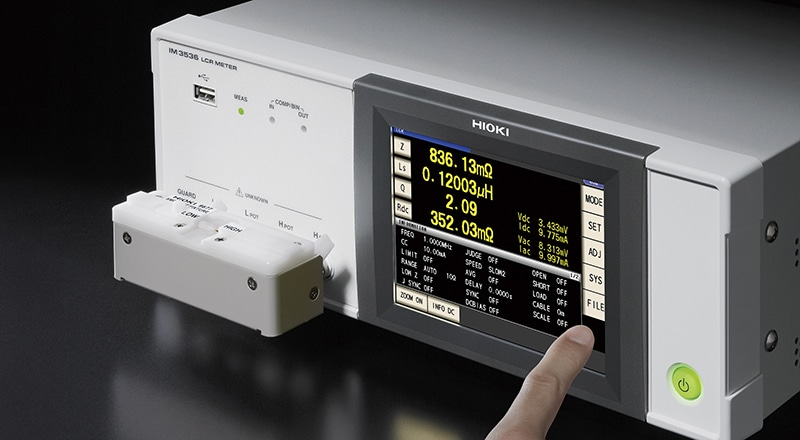This page addresses those questions by providing an easy-to-understand introduction to basic knowledge about impedance, how to use impedance meters, and precautions that should be borne in mind during measurement.

### What is impedance?

Some readers may not understand what impedance is. Impedance indicates resistance to an alternating current. It’s expressed using the symbol “Z” and the ohm (Ω) as a unit.

Larger numbers indicate greater resistance to the flow of electricity, while smaller numbers indicate less resistance. Impedance is a very important consideration in electric circuits.

•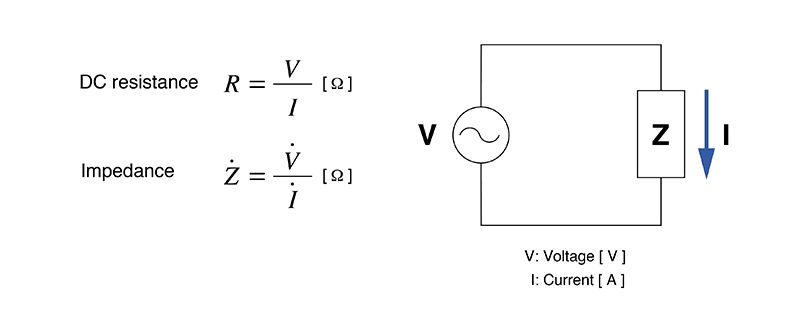### How does impedance differ from resistance in a direct current (DC) circuit?

Impedance is resistance, and it's even expressed using the same unit–ohms–as DC resistance. That said, DC resistance and impedance are not necessarily identical. On a general level, they describe the same concept, but AC impedance is more complex.

In a DC circuit, resistance (R) impedes the flow of current. In an AC circuit, coils (inductance, L) and capacitors (C) also impede the flow of current, in addition to resistance (R).

•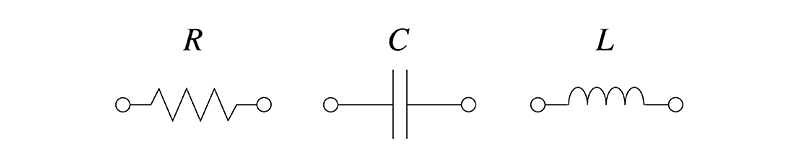### An impedance meter is necessary in order to measure impedance.

Since impedance is invisible, you’ll need a dedicated instrument in order to measure it. Instruments capable of measuring impedance include impedance meters, LCR meters, and impedance analyzers.

•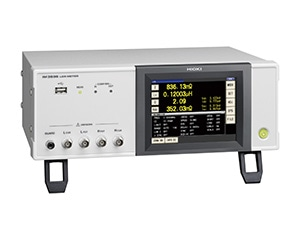LCR Meters
•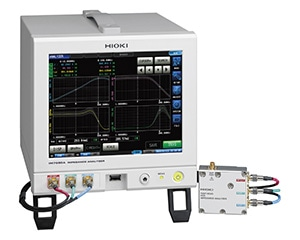Impedance Analyzers

There are multiple methods for measuring impedance, each with its own advantages and disadvantages. Consequently, the manner, range, and accuracy of your measurement will affect the instrument you choose.

The following table summarizes impedance measurement methods. You can use it to develop a general understanding of how impedance is measured.

Bridge methodHigh accuracy (about 0.1%)Is not well suited to high-speed measurement.
I-V methodCan measure samples that are grounded.Is susceptible to voltmeter effects in proportion to the magnitude of the impedance.
Automatically balanced bridge method
• Covers a broad range of frequencies (1 mHz to 100 MHz).
• Provides a broad impedance measurement range.

(Is used by many LCR meters.)

Cannot be used to measure high-frequency currents.
For frequencies in excess of several megahertz, you’ll need a complex I-V conversion circuit.
RF I-V method

Allows measurement of a broad range of impedance values with a high degree of accuracy (about 1%) and at high frequencies. (Compared to a network analyzer)

(Is used by high-frequency LCR meters.)

Is difficult to use for wideband measurement because the measurement frequency band is limited by the test head’s transformer.

### Precautions when using an impedance meter

•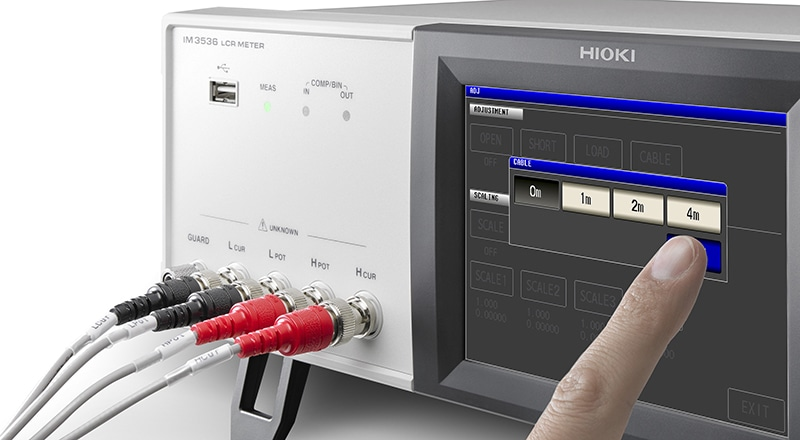Impedance measurement is an extremely delicate process, and measured values often fail to stabilize. Some potential causes of this issue are:

#### Variability caused by the components being measured

Impedance measurement is affected by individual differences among components being measured. In some cases, it’s impossible to reproduce measured values, even if measurements are made under the same conditions.

#### Parasitic components of the components being measured

In addition to the design values for resistance and reactance, components have parasitic components that cause variability in measured values. Even differences in the length of the leads connected to components and the distance between them can cause measured values to vary.

#### Variability caused by the conditions under which the instrument is being used

Measured values are affected by changes in the measurement environment, including the temperature of capacitors and inductors at the time of measurement, probe capacitance, and, when measuring high-impedance elements, external inductive noise. Furthermore, measurements can be affected by DC bias occurring in the circuit under measurement or the instrument.

Steps such as averaging multiple measurements are necessary in order to avoid variability in impedance measurement.

### Summary

There are various types of impedance meters, and they differ in measurement methods, frequency ranges, and other specifications. You’ll want to clarify the kind of measurements you need to make so that you can choose the right impedance meter. You’ll also need to take steps such as making repeated test measurements since various factors cause variability in impedance measurement.